# MO C–I–1 2018

An unknown number is divisible by just four numbers from the set {6, 15, 20, 21, 70}. Determine which ones.

Result

x = (Correct answer is: 6,15,21,70)### Step-by-step explanation: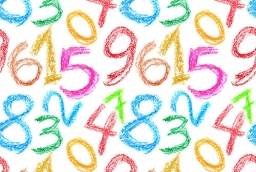Did you find an error or inaccuracy? Feel free to write us. Thank you!Tips to related online calculators
Do you want to calculate least common multiple two or more numbers?
Do you want to calculate greatest common divisor two or more numbers?

## Related math problems and questions:

• Lcm = 22 + gcdThe least common multiple of two numbers is 22 more than their greatest common divisor. Find these numbers.
• Gcd and lcm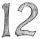Calculate the greatest common divisor and the least common multiple of numbers. a) 16 and 18 b) 24 and 22 c) 45 and 60 d) 36 and 30
• A number 5A number is divisible by 24, 25, and 120 if it is increased by 20. Find the number.
• Coloured numbersMussel wrote four different natural numbers with coloured markers: red, blue, green and yellow. When the red number divides by blue, it gets the green number as an incomplete proportion, and yellow represents the remainder after this division. When it div
• Apples and pearsMom divided 24 apples and 15 pears to children. Each child received the same number of apples and pears - same number as his siblings. How many apples (j=?) and pears (h=?) received each child?
• Lcm of three numbersWhat is the Lcm of 120 15 and 5
• DecomposeDecompose into primes and find the smallest common multiple n of (16,20) and the largest common divisor D of the pair of numbers (140,100)
• Pyramid Z8–I–6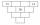Each brick of pyramid contains one number. Whenever possible, the number in each brick is lowest common multiple of two numbers of bricks lying directly above it. That number may be in the lowest brick? Determine all possibilities.
• LCD 2The least common denominator of 2/5, 1/2, and 3/4
• Trees in alleyThere are four trees in the alley between which the distances are 35m, 15m and 95m. Trees must be laid in the spaces so that the distance is the same and the maximum. How many trees will they put in and what will be the distance between them?
• Year 2018The product of the three positive numbers is 2018. What are the numbers?
• Length of a stringWhat is the smallest length of a string that we can cut into 18 equal parts and even 27 equal parts (in decimeters)?
• Counting numberWhat is the smallest counting number divisible by 2,5,7,8 and 15?
• Reminder and quotientThere are given the number C = 281, D = 201. Find the highest natural number S so that the C:S, D:S are with the remainder of 1,
• Paving - jointsWe are paving with rectangular pavement 18 cm × 24 cm was placed side by side in height in a row and in the second row in width etc. How many times will the joints meet at a distance 10 m?
• 9.A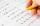9.A to attend more than 20 students but fewer than 40 students. A third of the pupils wrote a math test to mark 1, the sixth to mark 2, the ninth to mark 3. No one gets mark 4. How many students of class 9.A wrote a test to mark 5?
• Lcm 2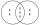Create the smallest possible number that is divisible by numbers 5,8,9,4,3